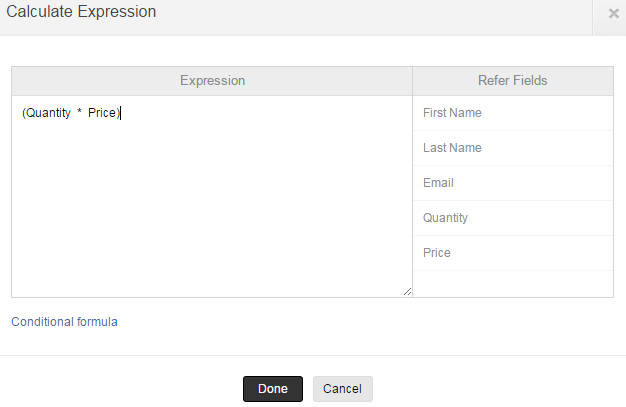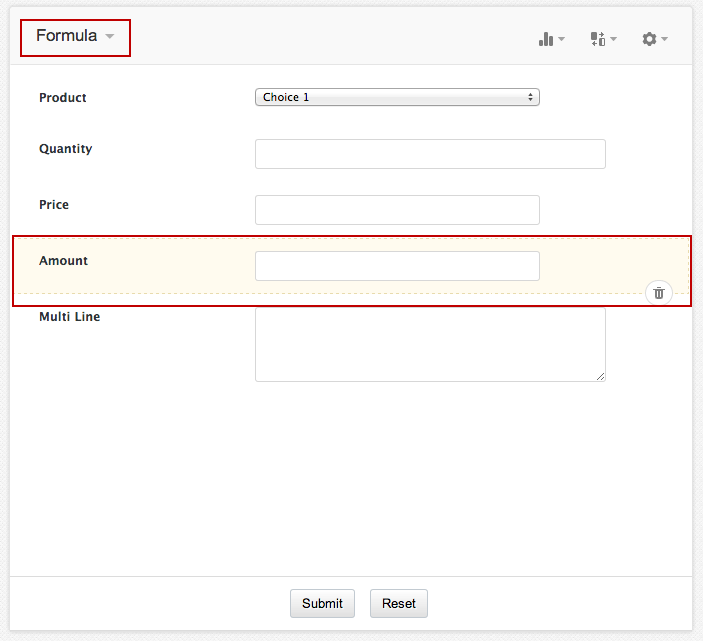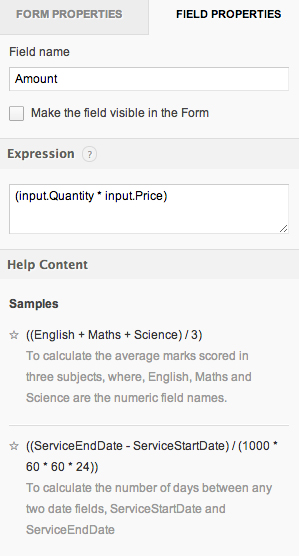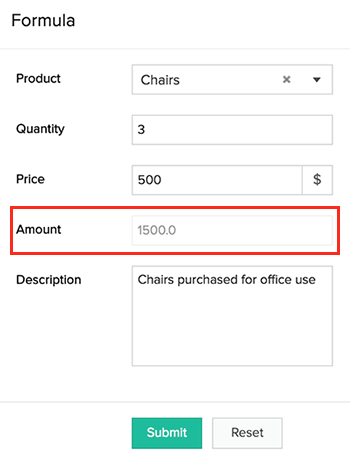# Configuring a Formula Field

## Overview

You can create fields that are calculated instead of being entered by the user. These fields are called the formula fields. When you create a formula field, you have to specify the formula expression based on which the value for this field is calculated. You can also display the formula value in the Form / Report or only in the Report, based on your requirement. By default, the formula value will be displayed only in the report.

Depending on the output of the formula, the value held by a formula field could be numeric values, text values, dates, and durations. The formula evaluation is nothing but expression evaluation and is explained in detail in Expressions.

Note

• Expressions containing null numeric values will not be executed as expected. Such expressions can be configured to have a null-check before evaluation.

## Steps in adding a formula field

1. Drag-n-drop the formula field type to the form editor area.
2. In the pop-up, specify the expression based on which the value for this field will be calculated. For example, in the "Bill Payment" form, we will add a formula field named Amount to calculate the Sales Amount based on the field values Quantity and Price. So, in the expression area, we will specify the expression Quantity * Price, where, Quantity and Rate are the field names. All the field names in the form are displayed below the expression area, for reference.3. In the Field Properties, specify the Field name  that will be displayed for this field, for example "Amount".4. By default, the formula field value will be displayed when you access the report. Select the check box option Make the field visible in the Form to display the formula value in the Form and the View.5. To edit the formula expression at any point of time, select the field and in the field properties make the necessary changes. The changes you make will be auto saved. If the option "Make the field visible in the Form" is selected, the Form will display the Amount field with value, when you access the Form.6. The formula field named Amount will be displayed along with its value in the Report.

Note:

• Each field that participates in a formula expression has a data type associated with it.The field in which you place the result of your formula also has a data type. All these data types and the Operators you use on them must be compatible. If you combine field types incorrectly, your formula won't work and Zoho Creator displays an "Error message" describing the data type mismatch.
• Deluge built-in functions and Deluge System Variables can be used in the formula expression.
• The expression can include fields in same form and fields in related forms (related via lookup and subform fields)
• The formula field's value in a record will be recalculated:
• The formula is modified
• User edits a record in the form that contains the formula field and modifies the value of any one of the fields participating in the formula. When the formula field refers to fields in related forms, editing records in the related forms will not cause recalculation of formula field's value.

## Sample Formula Expressions

1. To calculate the number of days between any two date fields, ServiceStartDate and ServiceEndDate

((ServiceEndDate - ServiceStartDate) / (1000 * 60 * 60 * 24))

2. To calculate the average marks scored in three subjects, where, English, Maths and Science are the numeric field names.

((English + Maths + Science) / 3)

3. To calculate the sum of all scores and display it as a percentage of total score, where score1, score2 and score3 are the numeric field names and round is a Deluge built-in function.

((score1 + score2 + score3) / 3 * 100).round(2)

4. To calculate the number of hours between any two date and time fields, To_Date and From_Date

((To_Date - From_Date) / (1000 * 60 * 60))

5. To create a unique code for each patient based on their DOB and Name combination, add a Formula field with formula expression as given below:

((((DOB.getMonth())) + Name) + (DOB.getDay())) + (DOB.getYear())

where,
- DOB.getMonth, returns a number in the range (1 -12), representing the number of the month of the year, on which the date occurs.
- DOB.getDay(), returns a number in the range (1 – 31), representing the number of the day of the month on which the date occurs.
- DOB.getYear(), returns a number representing the year of the date.
For example, if DOB is specified as 07-Sep-1994, with Name as “Henry”, the formula expression specified in the Code field, returns 9henry71994

6. To order the DOB field by month and day, add a formula field with expression,

((((((DOB.getMonth() / 100).round(2))) + "").getSuffix(".")) + "-") + ((((DOB.getDay() / 100).round(2))) + "").getSuffix(".")

where,

- DOB.getMonth(), returns a number in the range (1 -12), representing the number of the month of the year, on which the date occurs.
- DOB.getDay(), returns a number in the range (1 – 31), representing the number of the day of the month on which the date occurs.

The formula expression specified in the Order_DOB field, returns: [mm]-[dd]. It makes both the month and date, a two digit number. Otherwise ordering goes wrong like: 1, 10, 11, 12, 2, 3, etc.

7. To calculate the age of a person from a given DOB, add a Formula field and specify the formula expression as given below:

((zoho.currentdate - DOB) / (1000 * 3600 * 24 * 365)).round(2)

Note

If you use a Formula field, the calculated age can be seen only in the Report (i.e) the calculation is done after Form Submit. But, if you use a Number field the Age can be displayed in the Form itself before Submit.This is achieved by adding On user input script to the number field. Refer the sample application named Age Calculation which uses both the Formula Field (Age2) and a Number Field (Age), to calculate age.

## Conditional Expressions

In the following expression, when the boolean expression is true then variable is assigned with the value of expression1 else value of expression2 will be assigned

<variable> = if(<Boolean expression>, expression1, expression2)

Example 1:

The following formula expression can be used to check for null values in numeric fields. If the value of a numeric field is null, the value 0 is assigned, else the value of the number field is assigned.

(ifnull(input.number_field,0))

Example 2:

The following formula expression can be used to update the value of a field based on the value specified in another field. If the value of sales is greater than cost, the value "Profit" is assigned, else the value "Loss" is assigned.

if(input.sales > input.cost, "Profit","Loss")

Example 3:

The following formula expression can be used in the send mail task to send only those field values that are not null. This expression is used in the "Message" content of the send mail task. Here, Name and Email_1 are field names in the form.

if((input.Name != ""),"\nName : " + input.Name,"") + "<br />\n" + if((input.Email_1 != null),"\nEmail : " + input.Email_1,"") + "<br />"

For complex conditions, if statement can be used in the on add - on success block of Form Actions.

## Script Actions

You can use the Deluge round() function to round the result of your formula expression to two decimal places.To perform this action,

•     Go to edit mode and click on "Workflow" Tab
•     Select Field actions --> On User Input and enter the code.
•     Now access the application.
•     You can find the result of your formula expression rounded off according to the script entered in the workflow.

The syntax is given below:

(field1 + field2).round(Integer)

Example: (Decimal_field + Number_field).round(2)

In the above example the summation of decimal and number field is rounded off to two digits and the result is displayed.

## Related Links - Null check using conditional IF statement

Null check using conditional IF statement